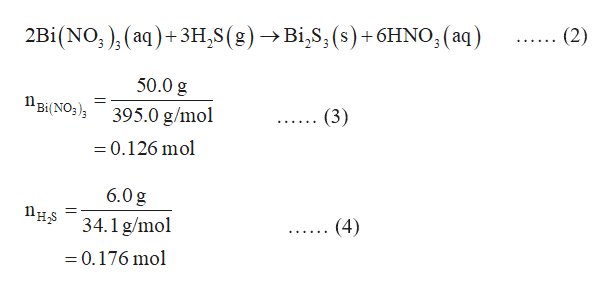Question
64 views
check_circle

Step 1

Limiting reagent is defined as a reactant which is present in a limited quantity in the chemical reaction. The reaction ceases after the consumption of the limiting reagent.

The theoretical yield of the product formed in the reaction is calculated by considering the number of moles of the limiting reagent.

The number of moles of a species is calculated by expression (1), in which, n is the number of moles, m is the mass, and M is the molar mass of the species.

Step 2

The given reaction is represented by equation (2).

The number of moles of bismuth nitrate and hydrosulfuric acid in the reaction are calculated in equations (3) and (4) respectively.help_outlineImage Transcriptionclose(2) (aq+3H2S (g) ->Bi,S, (s)+6HNO3(aq) 2Bi (NO, 50.0 g Bi(NO3) (3) 395.0 g/mol -0.126 mol 6.0 g 34.1 g/mol (4) =0.176 mol fullscreen
Step 3

In the reaction (2), 2 moles of bismuth nitrate reacts with 3 moles of hydrosulfuric acid.

This means 0.126 moles of bismuth nitrate reacts with 3/2 (0.126 mol) = 0.189 mol of hydrosulfuric acid. But the number of mol...

### Want to see the full answer?

See Solution

#### Want to see this answer and more?

Solutions are written by subject experts who are available 24/7. Questions are typically answered within 1 hour.*

See Solution
*Response times may vary by subject and question.
Tagged in

### Chemistry